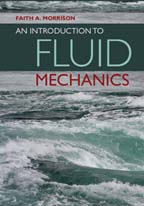| Michigan Tech Chemical Engineering | Michigan Tech Home PageFaith Morrison courses pageFaith Morrison landing pageDrMorrisonMTU YouTube |An Introduction to Fluid Mechanics

Cambridge University Press, 2013.

Also available on Amazon.com.

From the cover:  This is a modern and elegant introduction to engineering fluid mechanics enriched with numerous examples, exercises, and applications.  The goal of this textbook is to introduce the reader to the analysis of flows using the laws of physics and the language of mathematics.  The approach is rigorous, but mindful of the student.  Emphasis is on building engagement, competency, and problem-solving confidence that extends beyond a first fluids course.ISBN:  9781107003538
Publication date:  2013
940pages
Dimensions: 253 x 177 mm

Author:
Dr. Faith A. Morrison, Professor of Chemical Engineering

Michigan Technological University
1400 Townsend Drive
Houghton, MI 49931-1295
email fmorriso@mtu.eduThe Web Appendix (Appendix C and Appendix D) is available here.

The compact list of formulas from the inside covers is available here.

A handy sheet of unit conversions is available here

Errata for the Solution Manual is here.

End-of-Chapter Problems from Ch1, Ch2, Ch3, Ch4, Ch5, Ch6, Ch7, Ch8, Ch9, and Ch10.

Books by Faith Morrison:

Understanding Rheology, Oxford University Press, 2000
An Introduction to Fluid Mechanics, Cambridge University Press, 2013
Uncertainty Analysis for Engineers & Scientists:  A Practical Guide, Cambridge University Press, 2021

Course pages by Faith Morrison:

Errata for An Introduction to Fluid Mechanics
Please submit any errors you find to fmorriso@mtu.edu.

End Papers
Preface
Chapter 1
Chapter 2
Chapter 3
Chapter 4
Chapter 5
Chapter 6
Chapter 7
Chapter 8
Chapter 9
Chapter 10
Appendix A
Appendix B
Appendix C
Appendix D
References

Endpapers

Inside Front Cover:  The characteristic area in the Fanning Friction Factor denominator is 2*pi*R*L not pi*R^2

Preface
.

Chapter 1

p47 Chapter 1: Equation 1.133 should be 0.11 hp instead of 1.1 hp.

Chapter 2

Chapter 3

Chapter 4
Problem 4.14:  In the solution manual the density is taken to bee 13 g/cm3 instead of 13.6 g/cm3 as indicated in the text.  The correct answer is 720 Pa.

Chapter 5

Chapter 6

Chapter 7

page 569 av = 72.0 1/cm (not 720 1/cm)
page 591 Problem 7.33:  Instead of "isoceles" it should say "equilateral"

Chapter 8

Problem 8.19:  Should read: "Calculate the speed of the ball at impact, both with . . . "
page 675, Figure 8.32.  What is plotted is a dimensionlesss pressure.  The label is correct for potential flow pressure; for creeping flow pressure, the dimensionless pressure is (p-p_infty)/(\mu, v_\infty/2R).
page 734, Problem 12, the word "Stokes" should be replaced with, in the first instance, "creeping" and the second with "creeping flow".  Creeping flow is the same as Stokes flow, but it is better not to introduce this additional term.
page 735, Problem 8.19, "calculate the speed of the ball" should be "calculate the speed of the ball at impact"
page 736, Problem 31, line 2, "Figures 8.9 and 8.9" should read "Figures 8.9 and 8.24"
page 736, Problem 38, "1 inch" should be "1.0 inch" to reflect the correct number of significant figures.

Chapter 9

Chapter 10

page 870, Equation 10.97, p_2-p_1 should be p_1-p_2

Appendix A

Appendix B

Appendix C

Appendix D

References